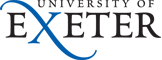Physics and Astronomy
Home Our Teaching Resources CDHW Feedback Technical-Info.html

## Technical Details About The Simulation

### The HTML Interface

The simulation is a fairly simple FORTRAN program front-ended by an HTML form. John Rowe's Metaform system processes the submitted form, identifying and substituting variables. It is this mechanism that updates the sentence about the set-point programme so that it describes the times and settings correctly. The browser reads the processed form and, by loading the graph, activates a second Perl script called Metagraph. Metagraph pipes parameter values from the form into the FORTRAN simulation code and passes the output to GNUPLOT which generates HPGL. This is converted to the gif format to be displayed by the browser.

### Frequency Domain Simulation

The frequency domain simulation PID-FD is straightforward; it calculates values for the frequency-domain magnitude and phase of the oven temperature using the defining equations for the oven and PID controller. FORTRAN's good feature, complex arithmetic, makes this very easy to do, about a dozen lines. This is increased to about 400 lines by the code that chooses nice ranges and tick mark positions so that it all fits on one graph that can be seen on a 13" monitor!

It is not a bug that causes the 'closed-loop' response curve sometimes to lie above the open-loop response. This can happen when the sensor-lag is non-zero because the graph shows how changing the set-point temperature affects To which is not the same as how changes in the temperature of the sensor affect To.

### Time Domain Simulation

If the noise density is zero the differential equations describing the oven are solved using the NAG routine D02CJF which is a variable-order variable-step Adams method. Small values of sensor lag (compared with the oven time constants) increase the calculation time so sensor-lag=0 is recognised and dealt with as a special case. The set-point ramp has had its corners replaced by part of a tanh() function to keep all derivatives continuous.

### Initial Conditions

The initial conditions are calculated on the assumption that the system has been running undisturbed for a very long time. For the On-Off controller the heater is off, the oven temperature is at the set-point and is falling at a rate found by setting the second derivative of To wrt time equal to zero. This was intended to be an approximation for test purposes but I have yet to see any evidence of a significant start-up transient resulting from it, so it'll do for now. For the PID controller things are simpler. The first derivative of To is zero, Terr=0 when the integral level is non-zero, or has the appropiate value when I=0. If I>0 then the integral of Terr wrt time is set to a value that will eliminate the static offset. This has the useful side-effect that if the static offset interfering with the scaling of a graph it can normally be eliminated by setting I to a very small value, such as 10-30, without affecting the dynamic response.

### Noise

One can't just add Gaussian noise from a random number generator to to this system and expect D02CBF, or similar adaptive integrators, to work. This is because they rely on the system of equations having derivatives that are continuous to high order. I've dealt with this problem here by generating a random spectrum for the noise and using the components of this to calculate Noise(time) and NoiseDerivative(time). The Fourier components of Noise(time) have random phase and frequency but unit amplitude. This trick has one undesirable property - it's slow to calculate. However, since the RMS noise can be estimated from the closed-loop amplitude response and the noise density, it should be possible to specify an absolute accuracy target for D02CBF which is slacker than the one used in the noise-free case and recover some of the speed that way.

### Problems

Most of the returned error messages are self-explanatory. Small, but non-zero values for the sensor lag significantly increase the CPU time needed for the simulation. In this context small means less than about 1% of shortest time constant associate with the oven, which is usually Ch*(Rho||Ro). Extremely small values of sensor lag cause solutions may become unreliable, which can be recognised because noise appears on the power signal W which is not evident when the sensor-lag is set to zero. Sensor lags less than 10-4 times the shortest time constant associated with the oven are rejected to try and avoid users ever seeing this problem.